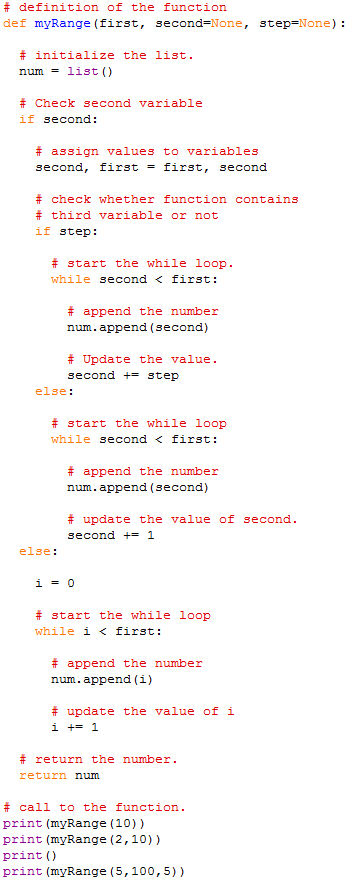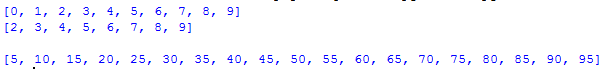# Question & Answer: Define and test a function myRange. This function should behave like Python’s standard rang…..

Define and test a function myRange. This function should behave like Python’s standard range function, with the required and optional arguments, but should return a list. Do not use the range function in your implementation!

Don't use plagiarized sources. Get Your Custom Essay on
Question & Answer: Define and test a function myRange. This function should behave like Python’s standard rang…..
GET AN ESSAY WRITTEN FOR YOU FROM AS LOW AS \$13/PAGE

Program Screenshots:

# Use python 3.5 or 3.6Sample Output:Code to Copy:

# Inundation of the program should be same as provided in above screenshots:

# Use python 3.5 or 3.6

# definition of the function
def myRange(first, second=None, step=None):

# initialize the list.
num = list()

# Check second variable
if second:

# assign values to variables
second, first = first, second

# check whether function contains
# third variable or not
if step:

# start the while loop.
while second < first:

# append the number
num.append(second)

# Update the value.
second += step
else:

# start the while loop
while second < first:

# append the number
num.append(second)

# update the value of second.
second += 1
else:

i = 0

# start the while loop
while i < first:

# append the number
num.append(i)

# update the value of i
i += 1

# return the number.
return num

# call to the function.
print(myRange(10))
print(myRange(2,10))
print()
print(myRange(5,100,5))